# Organic Chemistry I & II

Top Hat Intro Course - Organic Chemistry I & II is designed for instructors who want an active, dynamic, and understandable approach to support their own efforts in the classroom. This ever-evolving textbook includes auto-graded questions, videos and approachable language in order to make difficult concepts easier to understand and implement.

## What is a Top Hat Textbook?

Top Hat has reimagined the textbook – one that is designed to improve student readership through interactivity, is updated by a community of collaborating professors with the newest information, and accessed online from anywhere, at anytime.

• Top Hat Textbooks are built full of embedded videos, interactive timelines, charts, graphs, and video lessons from the authors themselves
• High-quality and affordable, at a significant fraction in cost vs traditional publisher textbooks

## Key features in this textbookBuilt-in assessment questions embedded throughout chapters so students can read a little, do a little, and test themselves to see what they know! “Keeping it Real”Sections connect theory to real-world examples and demonstrate the real-life applications of Organic Chemistry.

## Comparison of Organic Chemistry Textbooks

Consider adding Top Hat’s Organic Chemistry textbook to your upcoming course. We’ve put together a textbook comparison to make it easy for you in your upcoming evaluation.

### Top Hat

Steven Forsey, “Organic Chemistry”, Only one edition needed

### McGraw-Hill

Carey & Giuliano, “Organic Chemistry”, 10th Edition

### Wiley

Solomons et al., “Organic Chemistry”, 12th Edition

### Wiley

David R. Klein, “Organic Chemistry”, 3rd Edition

### Pricing

Average price of textbook across most common format

#### $219 Hardcover print text only ####$301

Hardcover print text only

#### $301 Hardcover print text only ### Always up-to-date content, constantly revised by community of professors Content meets standard for Introduction to Organic Chemistry course, and is updated with the latest content ### In-Book Interactivity Includes embedded multi-media files and integrated software to enhance visual presentation of concepts directly in textbook Only available with supplementary resources at additional cost Only available with supplementary resources at additional cost Only available with supplementary resources at additional cost ### Customizable Ability to revise, adjust and adapt content to meet needs of course and instructor ### All-in-one Platform Access to additional questions, test banks, and slides available within one platform ## Pricing Average price of textbook across most common format ### Top Hat Steven Forsey, “Organic Chemistry”, Only one edition needed #### Up to40-60%more affordable Lifetime access on any device ### McGraw-Hill Carey & Giuliano, “Organic Chemistry”, 10th Edition ####$219

Hardcover print text only

### Wiley

Solomons et al., “Organic Chemistry”, 12th Edition

#### $301 Hardcover print text only ### Wiley David R. Klein, “Organic Chemistry”, 3rd Edition ####$301

Hardcover print text only

## Always up-to-date content, constantly revised by community of professors

Constantly revised and updated by a community of professors with the latest content

### Top Hat

Steven Forsey, “Organic Chemistry”, Only one edition needed

### McGraw-Hill

Carey & Giuliano, “Organic Chemistry”, 10th Edition

### Wiley

Solomons et al., “Organic Chemistry”, 12th Edition

### Wiley

David R. Klein, “Organic Chemistry”, 3rd Edition

## In-book Interactivity

Includes embedded multi-media files and integrated software to enhance visual presentation of concepts directly in textbook

### Top Hat

Steven Forsey, “Organic Chemistry”, Only one edition needed

### McGraw-Hill

Carey & Giuliano, “Organic Chemistry”, 10th Edition

### Wiley

Solomons et al., “Organic Chemistry”, 12th Edition

### Wiley

David R. Klein, “Organic Chemistry”, 3rd Edition

## Customizable

Ability to revise, adjust and adapt content to meet needs of course and instructor

### Top Hat

Steven Forsey, “Organic Chemistry”, Only one edition needed

### McGraw-Hill

Carey & Giuliano, “Organic Chemistry”, 10th Edition

### Wiley

Solomons et al., “Organic Chemistry”, 12th Edition

### Wiley

David R. Klein, “Organic Chemistry”, 3rd Edition

## All-in-one Platform

### Top Hat

Steven Forsey, “Organic Chemistry”, Only one edition needed

### McGraw-Hill

Carey & Giuliano, “Organic Chemistry”, 10th Edition

### Wiley

Solomons et al., “Organic Chemistry”, 12th Edition

### Wiley

David R. Klein, “Organic Chemistry”, 3rd Edition

#### Dr. Steven Forsey, Ph.D.University of Waterloo

Steven Forsey is currently a Professor at University of Waterloo, teaching a variety of organic chemistry courses to Chemistry, Science, Chemical Engineering, Nanotechnology and distance education students. He received his Ph.D. (2004) for Synthetic Organic Chemistry from University of Waterloo, Ontario. He is a recipient of the Excellence of Science Teaching Award and has acted as the Teaching Fellow for the Department of Chemistry since 2016.

## Explore this textbook

Read the fully unlocked textbook below, and if you’re interested in learning more, get in touch to see how you can use this textbook in your course today.

# Chapter 1: Structure and BondingHave you ever wondered how geckos stick to walls so easily? As it turns out, their feet have millions of tiny hairs that attract the molecules in the wall through London dispersion forces. 

## Learning Objectives

• Understand the relationship between a wave function, quantum numbers and the three-dimensional image of an atomic orbital.
• Be able to write the valence shell electron configuration for main group atoms and ions.
• Understand the difference between ionic and covalent bonding.
• Be able to draw a valid Lewis structure for main group compounds, working from only the molecular formula and the electron configuration of the atoms.
• Be able to rank/assess the relative importance of a resonance structure by considering formal charges and the octet rule.
• Be able to predict the three-dimensional shape of a molecule or polyatomic ion from its Lewis structure using VSEPR theory.
• Be able to predict the hybrid orbitals an atom will use to make sigma bonds.
• Be able to use the Linear Combination of Atomic Orbitals (LCAO) method to generate molecular orbital energy diagrams for homonuclear diatomic molecules.
• Be able to distinguish between a polar covalent and non-polar covalent bond, using electronegativity values.
• Be able to identify the intermolecular forces that operate between molecules in a pure substance and in a mixture.
• Be able to rank pure substances according to their boiling points and melting points, based on the strength of the intermolecular forces they experience.
• Be able to predict if a substance will be soluble/miscible with non-polar/polar/hydrogen bonding solvents.
• Understand how intermolecular forces play a role in the function of surfactants/amphiphilic compounds.

## 1.0 Introduction

This chapter will help you review the most important information from general chemistry, as it pertains to organic chemistry. We will start by looking at how the electronic structure of individual atoms relates to bonding in molecules. Then we will learn how to predict the three-dimensional shapes of molecules. Finally, we will make the connection between molecular shape and composition and observable physical properties such as boiling points.

## 1.1 Atomic Orbitals

According to the Heisenberg Uncertainty Principle, the position of electrons has an uncertainty of at least h/4πmΔv (Equation 1.1).

Hence, the position of electrons is normally thought of in terms of regions of probability density. To find the energy and the probability density for an electron, one must solve the Schrödinger equation (Equation 1.2).

Applying the Hamiltonian operator (H) to the wave function (Ψ), which is a solution to Schrödinger’s wave equation, allows one to solve for the energy (E) of an electron residing in the atomic orbital described by the wave function. Squaring the wave function (Ψ2) describes the probability of finding an electron in a region around the nucleus of the atom. The three-dimensional plots of Ψ2 give the familiar atomic orbital shapes (Figure 1.1); the differential shading/coloring of the lobes of p and d orbitals represent regions where the wave function has a different mathematical sign (+ or -). The sign of the wave function has little physical significance for electrons in an atom, but the sign is important when atomic orbitals from different atoms overlap in order to share electrons and form a covalent bond. We will see this later in the chapter when we introduce Molecular Orbital Theory.

Each atomic orbital is described by three quantum numbers: n, l, and m. As a result of the quantized nature of energy at the atomic level, the quantum numbers are only allowed to have certain, discrete values (Table 1.1).

For single electron atoms and ions, the principal quantum number (n) determines the energy of an electron in the atomic orbital that corresponds to the most probable distance between the electron and the nucleus (i.e. orbital size). As the value of the principal quantum number increases, the maximum probability density moves farther from the nucleus, thereby increasing the energy of the electron.

The angular momentum quantum number (l) determines the shape of the atomic orbital. In multi-electron atoms and ions, the energy of an electron increases as the value of the angular momentum quantum number increases due to the effects of penetration and shielding. As the value of the angular momentum quantum number increases, the ability of an electron in the orbital to penetrate the inner shell electrons decreases, thereby raising the energy of the electron.

The magnetic quantum number (m) determines the directionality of the atomic orbital.

As described above, the principal quantum number n (n is an integer) plays a major role in determining the energy of an electron. For a single electron atom or ion, the energy of the electron is determined solely by the principal quantum number (Figure 1.2). The angular momentum quantum number l (l takes the values 0 to n-1) plays a role in determining the energy of an electron in a multi-electron atom or ion but not for a single electron system.  For s orbital, l =0; for  p orbital, l =1; for d orbital, l=2; and for f orbital, l =3. This is because shielding does not occur in these systems.Figure 1.2. Energy level diagrams for single electron (left) and multi-electron (right) atoms and ions (each dash represents one orbital)

Since organic molecules are held together by covalent bonds (i.e., shared pairs of electrons), it is important to know how electrons are distributed in atomic orbitals. In order to determine the electron configuration for an atom, we must consider the Aufbau principle, Hund’s rule and the Pauli exclusion principal. In most cases, these general guidelines will allow us to accurately determine how electrons populate atomic orbitals.

According to the Aufbau principle, electrons will populate orbitals so as to give the lowest total energy for the atom. Generally, this means that lower energy orbitals are populated before ( filled with two electrons) higher energy orbitals (Figure 1.3).

In addition to the three quantum numbers used to describe atomic orbitals, the spin quantum number (ms) is a characteristic of electrons. An electron may spin up (ms = +1/2) or spin down (ms = -1/2). According to Hund’s rule, electrons will populate orbitals in order to maximize the total electron spin. Additionally, placing two electrons in the same region of space (i.e. orbital) leads to repulsion and raises the energy of the electrons, thereby destabilizing the atom. Thus, within a subshell, electrons populate orbitals until each is half-filled, and only then may a second electron occupy an orbital (Figure 1.4).

The Pauli exclusion principle states that all electrons within an atom must have a unique set of four quantum numbers (all four quantum numbers cannot be the same for two electrons). As a result, only two electrons may reside in an atomic orbital and they must have opposite spins (Figure 1.5).

In order to determine the electron configuration for an atom, one must populate the orbitals with electrons—one for each proton in the nucleus—following the guidelines described above. For the first ten elements, this process is intuitive, but as the atomic number increases, it quickly becomes tedious.

A more expedient method for determining the electron configuration of an atom based on the periodic table is useful. The periodic table may be divided into blocks (Figure 1.6). In order to determine the electron configuration of an atom using the periodic table, start at hydrogen. Add one electron for each box on the periodic table until you arrive at the element of interest, keeping track as you fill each block. Notice that the row number corresponds to the principal quantum number for the s and p orbitals in each row. Notice also that the s-block is two columns wide because the s orbital can accommodate two electrons. Likewise, the p-block is six columns wide since there are three p orbitals and each orbital can accommodate two electrons for a total of six. As such, the d-block is ten columns wide, five d orbitals each accommodating two electrons for a total of ten.

Watch the video below to find out more about electron configurations.

Another benefit of the periodic table method for determining electron configurations is that it allows for the quick determination of the valence electron configuration for an element. Valence electrons are the outermost electrons of an atom and are directly involved in chemical bonding and reactions. In order to determine the valence electron configuration for main group elements (groups 1, 2, and 13-18), simply determine how the outermost s and p orbitals are populated (i.e. the s and p orbitals with the highest value for the principal quantum number). These are the electrons that will be used for bonding.

It becomes tedious for elements in row 3 and below to list all populated orbitals in order to write the electron configuration for an atom. A more concise method, and one that helps us focus on the valence electrons is to represent the core electrons by writing the symbol for the nearest noble gas with a lower maximum principal quantum number in brackets, and then to write the population for the outermost s and p orbitals (Figure 1.7). Electron configuration shown this way is called "shorthand electron configuration".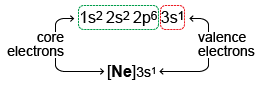Figure 1.7. Shorthand electron configuration of sodium (bottom) showing valence and core electrons.
Q1.1- Level 1

Match the elements with the correct valence electron configurations in the table below.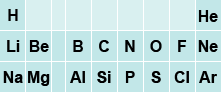Premise
Response
1

H

A

[He]2s$^1$

2

Be

B

1s$^1$

3

Al

C

[He]2s$^2$

4

N

D

[He]2s$^2$2p$^3$

5

S

E

[Ne]3s$^2$3p$^1$

F

[Ne]3s$^2$3p$^3$

G

[Ne]3s$^2$3p$^2$

H

[Ne]3s$^2$3p$^4$

Q1.2- Level 2

How many unpaired electrons are present in the ground state electronic configuration of a carbon atom?

Q1.3- Level 3

If $n$ = 3, which values of $ℓ$ are allowed?

A

0

B

1

C

2

D

3

E

4

Q1.4 - Level 2

If $n$ = 2, what type of orbitals are possible?

A

1s

B

2s

C

2p

D

3s

E

3p

F

3d

## 1.2 Chemical Bonding

Chemical bonds can be described very broadly as any force that causes a collection of atoms to behave as one entity rather than individual atoms. Within this description lie two extreme cases commonly encountered in organic chemistry: ionic bonding and covalent bonding.

### 1.2.1 Ionic Bonding

Ionic bonding occurs when a collection of cations and anions are held together as a result of the electrostatic attraction between the oppositely charged species. In ionic compounds, electrons are not shared between atoms; rather one or more electrons leave the valence orbital(s) of the nascent cation to reside in the valence orbital(s) of the newly formed anion (Figure 1.8).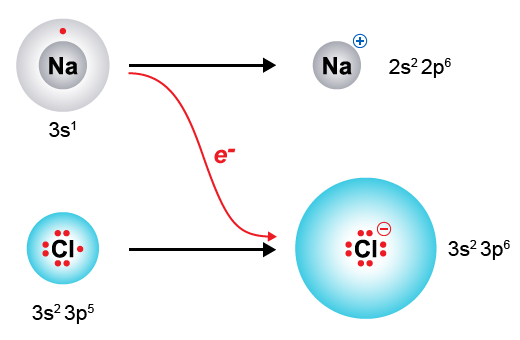Figure 1.8. Illustration of electron donation in ionic bonding between sodium and chlorine to make sodium chloride​

Covalent bonding results when two or more atoms come together to share electrons between nuclei more or less equally. The electrons in the internuclear region are simultaneously attracted to both nuclei, and allowed to occupy a larger region of space, thereby decreasing the electronic energy of the system, resulting in a stable covalent bond (Figure 1.9)

Most bonds are neither purely ionic nor purely covalent, but lie somewhere on a continuum. A simple method for classifying a compound as being held together by ionic or covalent bonds is to look at its constituent atoms. Compounds comprised of metals and non-metals are usually held together by ionic bonding and are classified as ionic compounds. Compounds comprised of only non-metallic elements are usually held together by covalent bonds, and are hence classified as covalent compounds. While this method works in many cases, it is not always accurate. For example, ammonium chloride (NH4Cl) is an ionic compound despite the fact that all of its atoms are non-metals; this compound is held together by the attraction between the ammonium cation and the chloride anion. Another example for which this simple method fails is in the case of organometallic compounds that have a metal–carbon bond; many of these bonds have significant covalent character.

Another method used to classify bonds as ionic or covalent relies on a property called electronegativity (X; Table 1.2), which may be thought of as the ability of an atom, when it is part of a compound, to pull electrons of its bonds with other atoms toward itself. The electronegativity difference (ΔX) between two atoms can be used to classify a bond as ionic, polar covalent, or non-polar covalent (Table 1.3).

In both polar covalent and non-polar covalent bonds, electrons are shared between two atoms. However, in the case of a polar covalent bond, the electrons are shared unequally, with the more electronegative element having a higher electron density, thereby leaving the less electronegative element electron deficient. Hence, in a polar covalent bond, the more electronegative element bears a partial negative charge (δ-) and the less electronegative element bears a partial positive charge (δ+) (Figure 1.10). Most of the bonds in organic molecules are either non-polar covalent or polar covalent. It is important to identify polar covalent bonds and atoms with significant partial positive or partial negative charge since these are often reactive sites.​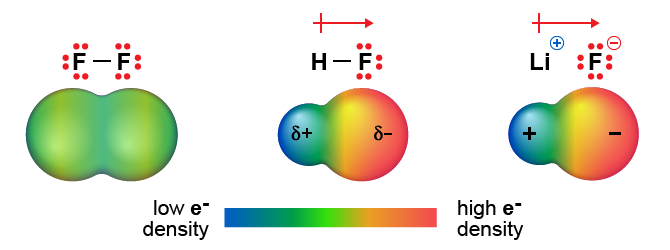Figure 1.10. From left to right, electron density of non-polar, polar covalent, and ionic compounds. The pointed end of the arrow denotes the part of the molecule with excess electron density. The crossed end of the arrow represents the part of the molecule that is electron deficient.​

Q1.5 - Level 3

Consider the molecular formulas below and classify the following compounds as ionic, covalent, or a mixture of ionic and covalent.

Premise
Response
1

CaCO$_3$

A

Covalent

2

CF$_4$

B

Ionic

3

NH$_4$NO$_3$

C

Covalent

4

SOCl$_2$

D

Mixture

5

CH$_3$NO

E

Ionic

6

LiF

F

Mixture

G

Mixture

H

Covalent

I

Ionic

Q1.6 - Level 1

Use the electronegativity values from Table 1.2 (below), to determine if the following bonds are likely to be non-polar covalent, polar covalent, or ionic. Match each bond to its appropriate classification.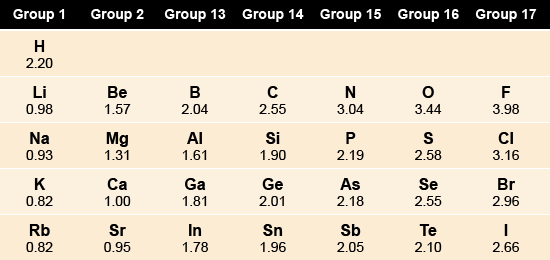Premise
Response
1

Li–C

A

Polar covalent

2

H-C

B

Non-polar covalent

3

O-C

C

Ionic

4

N-C

D

Non-polar covalent

E

Ionic

F

Polar covalent

Q1.7 - Level 1

Rank the following bonds from most polar to least polar.

A

C-N

B

C-F

C

C-O

### 1.2.2 Covalent Bonding

As stated above, covalent bonding results when atoms come together to share electrons. The driving force for covalent bond formation is partially the fact that electrons residing between two nuclei are attracted to both nuclei simultaneously. Since electrostatic attraction leads to a decrease in electronic energy, sharing electrons in a covalent bond results in a net stabilization of the system comprised of the atomic nuclei and their electrons (Figure 1.11).

### 1.2.2A Valence Bond Theory

In the early 20th century, G.N. Lewis and others noticed that most main group elements would gain, lose, or share electrons in order to achieve a full valence shell of eight electrons. This became known as the octet rule. Main group elements have four total valence orbitals—one s orbital and three p orbitals—and can therefore accommodate eight electrons in their valence shell. In covalent compounds, atoms seek to share electrons so that all atoms in the molecule have a filled valence shell. Making use of this simple idea, one can predict, with surprising accuracy, the two-dimensional structure of many main group compounds.

### 1.2.2B Lewis Structures

The following steps will allow you to predict the two-dimensional structure of a molecule and the bond order of each bond working from only the molecular formula:

Step 1: Determine the total number of valence electrons. These will be used to form bonds and lone pairs (Figure 1.12).

• For a molecule, this will be the sum of all valence electrons of all the atoms in the formula
• For a polyatomic anion, add one electron for each negative charge to the sum of all valence electrons for every atom in the formula
• For a polyatomic cation, subtract one electron for each positive charge from the sum of all valence electrons for every atom in the formula

Step 2: Find the central atom (Figure 1.13).

• The central atom usually has the lowest electronegativity
• Hydrogen is never the central atom

Step 3: Draw a single bond between the central atom and each peripheral atom (Figure 1.14).

• Each bond is represented with a line and counts as two electrons
• For row 2 elements, you cannot exceed an octet of electrons (no more than four bonds to the central atom)
• For row 3 elements and below, you may exceed an octet of electrons by invoking “low-lying” empty d orbitals. It is rare to exceed six bonds to the central atom

Step 4: Add lone pairs to each of the peripheral atoms until each peripheral atom has an octet of electrons, or until you run out of electrons (Figure 1.15).

Step 5: If electrons remain, add them as non-bonding electrons to the central atom.

• Non-bonding electrons are represented by dots (•) and are typically observed as pairs of electrons (••)
• If you began with an odd number of electrons, the unpaired electron will usually be localized on the least electronegative element (e.g. N in nitric oxide; Figure 1.15)
• The total number of electrons surrounding a row 2 element (bonding and non-bonding) may not exceed eight
• The total number of electrons surrounding a row 3 or lower element (bonding and non-bonding) may exceed eight but rarely exceeds twelve

Step 6: If the central atom has less than an octet of electrons, use one or more lone pairs on the least electronegative peripheral atoms to make multiple bonds until the central atom achieves an octet (Figure 1.16). In cases where an odd number of valence electrons is present (e.g. NO), it is not possible to achieve an octet; in general, the less electronegative atom will be electron deficient in these species.Figure 1.16. Converting lone pairs to π bonds in order to satisfy the octet rule​

Step 7: Calculate the formal charge on each element using Equation 1.3.

Formal charge = # valence e- in neutral element - [# non-bonding e- + $\frac12$ (# bonding e-)]

Equation 1.3

Formal charge is based on the premise that bonding electrons are shared equally; if a bond were to break, it would do so homolytically. Formal charge is calculated by taking the difference between the number of valence electrons an atom has when it is not part of a molecule, and the number of electrons an atom can count for itself when it is part of a molecule. The concept of formal charge is depicted below.

Consider the nitrogen atom of this molecule. A nitrogen atom has a valence electron configuration of 2s22p3; there are five electrons in nitrogen’s valence shell. In this structure, nitrogen can only count four electrons for itself—one from each bond (half of the total electrons of its bonds). Hence, in this molecule, the formal charge on nitrogen is 5 – 4 = +1.

The video linked below is a useful tutorial for calculating formal charge in organic molecules, and also reviews important concepts regarding geometry, and bonding from general chemistry.

Here are a few notes on formal charges:

• For most organic molecules, the formal charge on each atom is zero
• The most likely structure is usually that in which the formal charges have been minimized
• If formal charges persist, negative charges should be on more electronegative elements, and positive charges should be on less electronegative elements
• If two atoms with opposite formal charges are connected, this separation of formal charge should be eliminated by making multiple bonds. Please note that this structural refinement does not supersede the octet rule for row 2 elements (Figure 1.18)
• Structures in which two atoms with identical formal charges are connected are unlikely to be an accurate representation of the molecule or polyatomic ion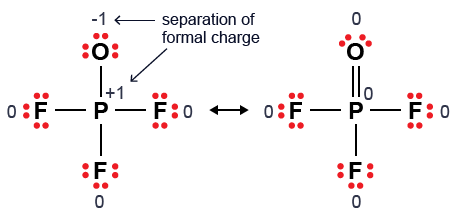Figure 1.18. Minimizing the separation of formal charge by forming multiple bonds.​

The rules above will allow you to accurately predict the two-dimensional structures and bond orders for many main group compounds. Organic molecules are mainly comprised of row 2 elements and hydrogen, but may contain repetitive chain or ring motifs. Therefore, Step 2 may not be applicable in some cases (Figure 1.19). As the number of atoms in the molecule increases, the number of possible, valid Lewis structures—constitutional isomers—increases as well. Keep these general guidelines in mind when proposing structures for organic molecules:

• Carbon atoms are usually the central atom in small organic molecules, and serve as the repeating unit in chain and ring structures
• Formal charges in organic molecules are usually zero, and rarely exceed ±1
• For neutral organic molecules, note the following:
• Carbon makes four total bonds (never more!)
• Nitrogen makes three total bonds
• Oxygen makes two total bonds
• Halogens make one total bond
• Hydrogen makes one total bond
• Row 2 elements cannot exceed an octet of electrons

Note that all of these guidelines are followed by the structures of molecules in Figure 1.19.

The table below shows the general structure of charged and neutral boron, carbon, nitrogen, oxygen and halogen organic molecules. In organic chemistry, it is very important that you are able to identify the charge on atoms. It is part of the language.

Note: Only carbon is allowed to have a positive charge and not have a full octet. A nitrogen, oxygen and halogen with a positive charge must have a full octet.

Q1.8 - Level 1

Assign formal charges to the atoms in the Lewis structure below.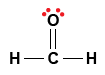A

(-2)

B

(-1)

C

(0)

D

(+1)

E

(+2)

Q1.9 - Level 1

Assign formal charges to the atoms in the Lewis structure below.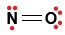A

(-2)

B

(-1)

C

(0)

D

(+1)

E

(+2)

Q1.10 - Level 1

Assign formal charges to the atoms in the Lewis structure below.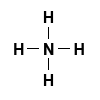Premise
Response
1

N

A

(0)

2

H

B

(-2)

C

(+2)

D

(-1)

E

(+1)

Q1.11 - Level 1

Assign formal charges to the Carbon in the Lewis structure below.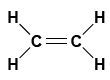A

(-2)

B

(-1)

C

(0)

D

(+1)

E

(+2)

### 1.2.2C Bond-Line Drawings

Many important organic molecules, especially biologically active organic molecules, have complex structures that are difficult to depict using Lewis structures, given the spatial constraints imposed by using letters to represent atoms of different elements. In order to address this difficulty, chemists have developed the bond-line drawing convention that allows organic molecules to be drawn expediently, while minimizing the use of space. The bond-line method makes use of the fact that most of the atoms in organic molecules are carbon and hydrogen. In this method, carbon atoms are represented by the end-points and intersections of lines. It is assumed that each carbon makes four bonds, any bonds not explicitly drawn are assumed to be between carbon and hydrogen.

Perhaps the simplest way to learn how to use the bond-line method is to convert a bond line structure to an expanded Lewis structure. Consider the molecule in Figure 1.20.

Step 1: Locate all of the carbon atoms. Recall that these are at the intersections and end-points of lines.

Step 2: Add in hydrogens bound to carbon atoms. Unless there is a charge associated with a carbon atom, you should assume it has four bonds. All bonds not explicitly drawn are assumed to be between carbon and hydrogen.

Step 3: Add in lone pairs. Unless there is a charge on an atom, all atoms should be assumed to have an octet.

Step 4: If charges are present on a carbon atom, you will need to remove one hydrogen atom. In the case of a positively charged carbon, this is all you need to do. In the case of a negatively charged carbon, you will need to recognize that there is a lone pair of electrons localized on that carbon atom.

Q1.12 - Level 2

What is the molecular formula for the compound below?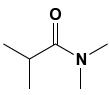A

C$_5$H$_{12}$NO

B

C$_5$H$_{13}$NO

C

C$_6$H$_{12}$NO

D

C$_6$